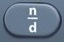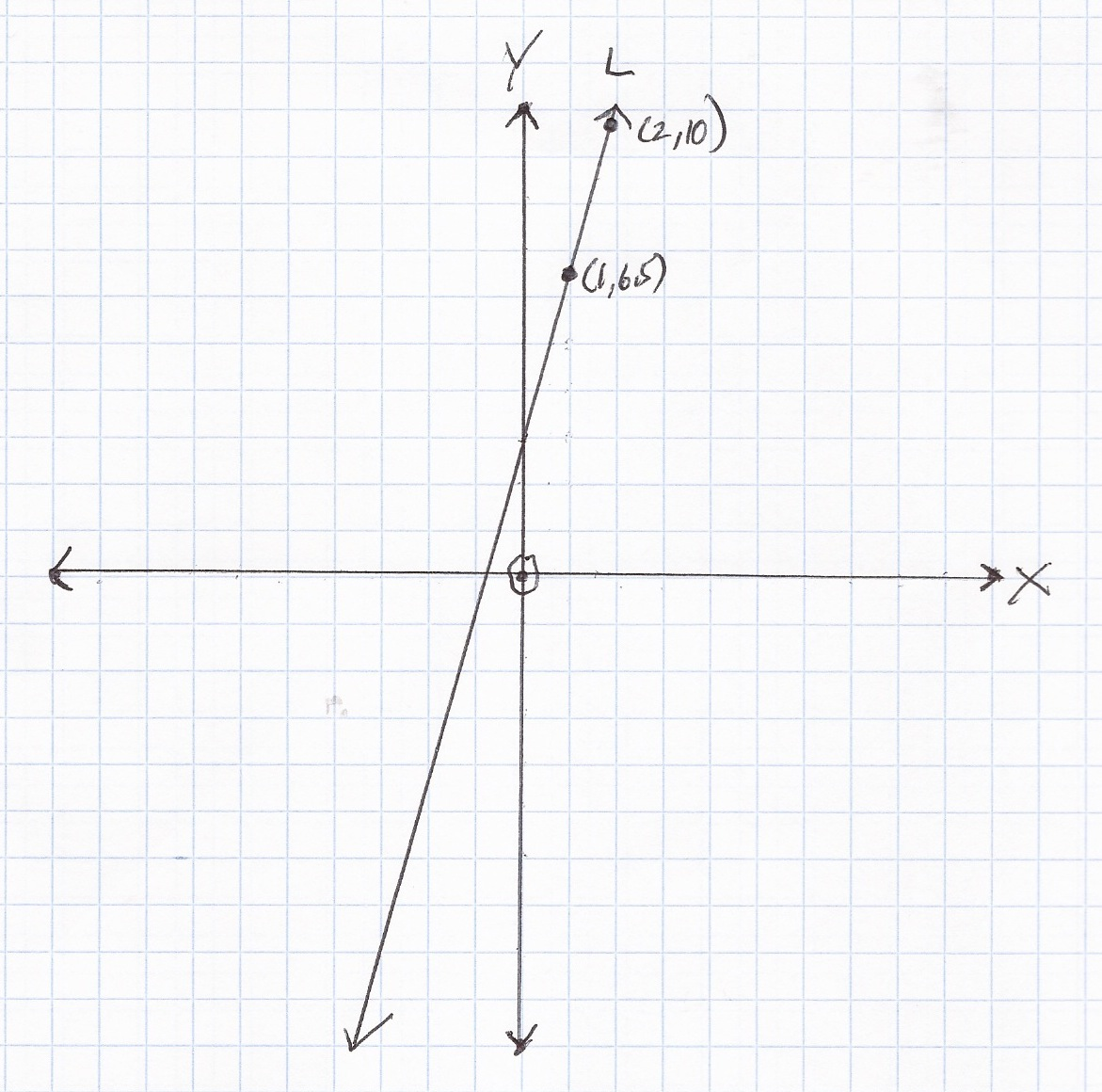# Slope-InterceptBasics
(0, b) = y-intercept = slope-intercept = point where line crosses y-axis

A question involving slope-intercept will often require you to use two points to calculate the slope and y-intercept on the way to writing the equation of a line.

Although plotting points and a line on an x-y coordinate plane or grid can help visualize the process, a question involving slope-intercept can often be solved without graphing.

Formulas (
Mathematics Formula Sheet)
slope-intercept form of the equation of a line
y = mx + b

slope of a line
m =$\bf\displaystyle\frac{{{y}_{2}}-{{y}_{1}}}{{{x}_{2}}-{{x}_{1}}}$

Formulas (NOT on Mathematics Formula Sheet)
slope-intercept b
b = y – mx

Question
Using the points (1, 4) and (3, 9) in the graph below, write the slope-intercept equation for Line L.  The slope and y-intercept should be in decimal form.y = 2.5x + 1.5

(x1, y1) = (1, 4)
(x2, y2) = (3, 9)

slope of a line
m =$\bf\displaystyle\frac{{{y}_{2}}-{{y}_{1}}}{{{x}_{2}}-{{x}_{1}}}$

m =$\bf\displaystyle\frac{9-4}{3-1}$ =$\bf\displaystyle\frac{5}{2}$ = 2.5 after toggle

slope-intercept b
b = y – mx

(x1, y1) or (x2, y2) can be used to calculate b; in this case, (x1, y1) = (1, 4) will be used:
slope-intercept b
b = y – mx = 4 – 2.5 × 1 = 1.5

slope-intercept form of the equation of a line
y = mx + b

y = 2.5x + 1.5

Input Display Commentblinker clears screen9 – 43 – 1$\bf\displaystyle\frac{9-4}{3-1}$ m =$\bf\displaystyle\frac{{{y}_{2}}-{{y}_{1}}}{{{x}_{2}}-{{x}_{1}}}$$\bf\displaystyle\frac{5}{2}$2.5 m
(Toggle)
4 – 2.5 × 1 4-2.5*1 b = y – mx1.5 b
###### Slope-Intercept

Question
Using the points (-2, 3) and (-4, 7) in the graph below, write the slope-intercept equation for Line L.  The slope and y-intercept should be in decimal form.y = -2x – 1

(x1, y1) = (-2, 3)
(x2, y2) = (-4, 7)

slope of a line
m =$\bf\displaystyle\frac{{{y}_{2}}-{{y}_{1}}}{{{x}_{2}}-{{x}_{1}}}$

m =$\bf\displaystyle\frac{7-3}{-4- -2}$ =$\bf\displaystyle\frac{4}{-2}$ = -2

slope-intercept b
b = y – mx

(x1, y1) or (x2, y2) can be used to calculate b; in this case, (x1, y1) = (-2, 3) will be used:
b = y – mx = 3 – -2 × -2 = -1

slope-intercept form of the equation of a line
y = mx + b

y = -2x – 1

Input Display Commentblinker clears screen7 – 3-4 – -2$\bf\displaystyle\frac{7-3}{-4- -2}$ m =$\bf\displaystyle\frac{{{y}_{2}}-{{y}_{1}}}{{{x}_{2}}-{{x}_{1}}}$-2 m
3 – -2 × -2 3- -2* -2 b = y – mx-1 b
###### Slope-Intercept

Question
Without a graph, write the slope-intercept equation for the line passing through points (3, 7) and (5, 9.5).  The slope and y-intercept should be in decimal form.

y = 1.25x + 3.25

(x1, y1) = (3, 7)
(x2, y2) = (5, 9.5)

slope of a line
m =$\bf\displaystyle\frac{{{y}_{2}}-{{y}_{1}}}{{{x}_{2}}-{{x}_{1}}}$

m =$\bf\displaystyle\frac{9.5-7}{5-3}$ = 1.25

slope-intercept b
b = y – mx

(x1, y1) or (x2, y2) can be used to calculate b; in this case, (x1, y1) = (3, 7) will be used:
b = y – mx = 7 – 1.25 × 3 = 3.25

slope-intercept form of the equation of a line
y = mx + b

y = 1.25x + 3.25

Input Display Commentblinker clears screen9.5 – 75 – 3$\bf\displaystyle\frac{9.5-7}{5-3}$ m =$\bf\displaystyle\frac{{{y}_{2}}-{{y}_{1}}}{{{x}_{2}}-{{x}_{1}}}$1.25 m
7 – 1.25 × 3 7-1.25*3 b = y – mx3.25 b
###### Slope-Intercept

Question
Without a graph, write the slope-intercept equation for the line passing through points (-3, 6) and (-5, 8.5).  The slope and y-intercept should be in decimal form.

y = -1.25x + 2.25

(x1, y1) = (-3, 6)
(x2, y2) = (-5, 8.5)

slope of a line
m =$\bf\displaystyle\frac{{{y}_{2}}-{{y}_{1}}}{{{x}_{2}}-{{x}_{1}}}$

m =$\bf\displaystyle\frac{8.5-6}{-5- -3}$ = -1.25

slope-intercept b
b = y – mx

(x1, y1) or (x2, y2) can be used to calculate b; in this case, (x1, y1) = (-3, 6) will be used:
b = y – mx = 6 – 1.25 × -3 = 2.25

slope-intercept form of the equation of a line
y = mx + b

y = -1.25x + 2.25

Input Display Commentblinker clears screen8.5 – 6-5 – -3$\bf\displaystyle\frac{8.5-6}{-5- -3}$ m =$\bf\displaystyle\frac{{{y}_{2}}-{{y}_{1}}}{{{x}_{2}}-{{x}_{1}}}$-1.25 m
6 – -1.25 × -3 6- -1.25* -3 b = y – mx2.25 b
###### Slope-Intercept

Question
The following table shows the age in years and height in inches of a boy and a girl.

Write the slope-intercept equation for the line passing through the boy’s growth points at (2, 34.00) and (4, 40.00).

 Boy Girl Age (yr) Height (in) Age (yr) Height (in) 2 34.00 2 33.50 3 37.50 3 37.00 4 40.00 4 39.50 5 43.00 5 42.25 6 45.25 6 45.00 7 48.00 7 48.00 8 50.25 8 50.00 9 52.50 9 52.50 10 54.50 10 54.25

y = 3x + 28

(x1, y1) = (2, 34.00)

(x2, y2) = (4, 40.00)

slope of a line
m =$\bf\displaystyle\frac{{{y}_{2}}-{{y}_{1}}}{{{x}_{2}}-{{x}_{1}}}$

m =$\bf\displaystyle\frac{40.00-34.00}{4-2}$ = 3

slope-intercept b
b = y – mx

(x1, y1) or (x2, y2) can be used to calculate b; in this case, (x1, y1) = (2, 34.00) will be used:
b = y – mx = 34.00 – 3 × 2 = 28

slope-intercept form of the equation of a line
y = mx + b

y = 3x + 28

Input Display Commentblinker clears screen40.00 – 34.004 – 2$\bf\displaystyle\frac{40.00-34.00}{4-2}$ m =$\bf\displaystyle\frac{{{y}_{2}}-{{y}_{1}}}{{{x}_{2}}-{{x}_{1}}}$3 m
34.00 – 3 × 2 34.00-3*2 b = y – mx28 b
###### Slope-Intercept

Question
The following table shows the growth of an investment over time.

 Time (years) Value (dollars) 2 100 4 120 6 140 8 160 10 180

Based on the data in the table, which of the following slope-intercept equations represents the growth of an investment over time, where x is the year and y is the dollar value?
A.  y = -10x + 80
B.  y = 10x – 80
C.  y = -10x – 80
D.  y = 10x + 80

D.  y = 10x + 80

Any two points in the data table will do; in this case, the following points will be used:

(x1, y1) = Point (2, 100)
(x2, y2) = Point (4, 120)

slope of a line
m =$\bf\displaystyle\frac{{{y}_{2}}-{{y}_{1}}}{{{x}_{2}}-{{x}_{1}}}$

m =$\bf\displaystyle\frac{120-100}{4-2}$ = 10

slope-intercept b
b = y – mx

(x1, y1) or (x2, y2) can be used to calculate b; in this case, (x1, y1) = (2, 100) will be used:
b = y – mx = 100 – 10 × 2 = 80

slope-intercept form of the equation of a line
y = mx + b

y = 10x + 80

Input Display Commentblinker clears screen120 – 1004 – 2$\bf\displaystyle\frac{120-100}{4-2}$ m =$\bf\displaystyle\frac{{{y}_{2}}-{{y}_{1}}}{{{x}_{2}}-{{x}_{1}}}$10 m
100 – 10 × 2 100-10*2 b = y – mx80 b
###### Slope-Intercept

Question

Bob planted some prairie grass to beautify a corner of his yard.  After 3 weeks, the grass was 12 inches tall.  After 7 weeks, the grass was 24 inches tall.  If it grows at the same rate, how tall will the grass be at week 10?

33 inches

(x1, y1) = (3, 12)
(x2, y2) = (7, 24)

slope of a line
m =$\bf\displaystyle\frac{{{y}_{2}}-{{y}_{1}}}{{{x}_{2}}-{{x}_{1}}}$

m =$\bf\displaystyle\frac{24-12}{7-3}$ = 3

slope-intercept b
b = y – mx

(x1, y1) or (x2, y2) can be used to calculate b; in this case, (x1, y1) = (3, 12) will be used:
b = y – mx = 12 – 3 × 3 = 3

slope-intercept form of the equation of a line
y = mx + b

y = 3x + 3

See Plug-In.
Plug in 10 for x.
y = 3 × 10 + 3 = 33

Input Display Commentblinker clears screen24 – 127 – 3$\bf\displaystyle\frac{24-12}{7-3}$ m =$\bf\displaystyle\frac{{{y}_{2}}-{{y}_{1}}}{{{x}_{2}}-{{x}_{1}}}$3 m
12 – 3 × 3 12-3*3 b = y – mx3 b
3 × 10 + 3 3*10+3 y = mx + b
Plug in 10 for x.33 Answer
###### Slope-Intercept

Practice – Questions
1.  Using the points (1, 6.5) and (2, 10) in the graph below, write the slope-intercept equation for Line L.  The slope and y-intercept should be in decimal form.2.  Without a graph, write the slope-intercept equation for the line passing through points (-4, 8) and (-8, 13).  The slope and y-intercept should be in decimal form.

3.  The following table shows the age in years and height in inches of a boy and a girl.

Write the slope-intercept equation for the line passing through the girl’s growth points at (3, 37.00) and (7, 48.00).  The slope and y-intercept should be in decimal form.

 Boy Girl Age (yr) Height (in) Age (yr( Height (in) 2 34.00 2 33.50 3 37.50 3 37.00 4 40.00 4 39.50 5 43.00 5 42.25 6 45.25 6 45.00 7 48.00 7 48.00 8 50.25 8 50.00 9 52.50 9 52.50 10 54.50 10 54.25

4.  The following table shows the growth of an investment over time.

 Time (years) Value (dollars) 3 250 5 500 7 750 9 1000 11 1250

Based on the data in the table, which of the following slope-intercept equations represents the growth of an investment over time, where x is the year and y is the dollar value?
A.  y = -125x – 125
B.  y = -125x + 125
C.  y = 125x – 125
D.  y = 125x + 125

5.  The following table shows the depreciation (loss in value) of an automobile over time.

 Age (years) Value (dollars) 0 25000 2 18000 4 11000 6 4000

Based on the data in the table, which of the following slope-intercept equations represents the depreciation (loss in value) of an automobile over time, where x is the age in years and y is the dollar value?
A.  y = 3500x – 25000
B.  y = -3500x + 25000
C.  y = -3500x – 25000
D.  y = 3500x + 25000

6.  Bob planted some prairie grass to beautify a corner of his yard.  After 4 weeks, the grass was 18 inches tall.  After 8 weeks, the grass was 48 inches tall.  If it grows at the same rate, how tall will the grass be at week 12?

7.  Betty sent 2 text messages at 4:00 AM and 7 text messages at 6:00 AM.  If Betty increases her text message output at the same rate, how many texts will she send at 10 AM?

8.  Bob spent $3.00 on popcorn at 5:00 PM and$4.50 on popcorn at 7:00 PM.  If Bob increases his popcorn expenditures at the same rate, how much will he spend on popcorn at 10:00 PM?

9. The following table shows the approximate weight in kilograms (kg) over the first ten weeks of a pig’s life.

 Age (weeks) Weight (kg) 0 1 1 2 2 3 3 5 4 8 5 10 6 15 7 18 8 20 9 25 10 30

Using the table’s data points of (0, 1) and (2, 3), write a slope-intercept equation for the pig’s gain in weight, where x is the age in weeks and y is the weight in kg.

10.  With reference to Question 9, use the table’s data points of (8, 20) and (10, 30) to write a slope-intercept equation for the pig’s gain in weight, where x is the age in weeks and y is the weight in kg.

1.  y = 3.5x + 3

2.  y = -1.25x + 3

3.  y = 2.75x + 28.75

4.  C.  y = 125x – 125

5.  B.  y = -3500x + 25000

6.  78 inches

7.  17

8.  \$6.75

9.  y = x + 1

10.  y = 5x – 20